The ARIMA Procedure
 OUTEST= Data Set

PROC ARIMA writes the parameter estimates for a model to an output data set when the OUTEST= option is specified in the ESTIMATE statement. The OUTEST= data set contains the following:

• the BY variables

• _MODLABEL_, a character variable that contains the model label, if it is provided by using the label option in the ESTIMATE statement (otherwise this variable is not created).

• _NAME_, a character variable that contains the name of the parameter for the covariance or correlation observations or is blank for the observations that contain the parameter estimates. (This variable is not created if neither OUTCOV nor OUTCORR is specified.)

• _TYPE_, a character variable that identifies the type of observation. A description of the _TYPE_ variable values is given below.

• variables for model parameters

The variables for the model parameters are named as follows:

ERRORVAR

This numeric variable contains the variance estimate. The _TYPE_=EST observation for this variable contains the estimated error variance, and the remaining observations are missing.

MU

This numeric variable contains values for the mean parameter for the model. (This variable is not created if NOCONSTANT is specified.)

MAj _k

These numeric variables contain values for the moving-average parameters. The variables for moving-average parameters are named MAj _k, where j is the factor-number and k is the index of the parameter within a factor.

ARj _k

These numeric variables contain values for the autoregressive parameters. The variables for autoregressive parameters are named ARj _k, where j is the factor number and k is the index of the parameter within a factor.

Ij _k

These variables contain values for the transfer function parameters. Variables for transfer function parameters are named Ij _k, where j is the number of the INPUT variable associated with the transfer function component and k is the number of the parameter for the particular INPUT variable. INPUT variables are numbered according to the order in which they appear in the INPUT= list.

_STATUS_

This variable describes the convergence status of the model. A value of 0_CONVERGED indicates that the model converged.

The value of the _TYPE_ variable for each observation indicates the kind of value contained in the variables for model parameters for the observation. The OUTEST= data set contains observations with the following _TYPE_ values:

EST

The observation contains parameter estimates.

STD

The observation contains approximate standard errors of the estimates.

CORR

The observation contains correlations of the estimates. OUTCORR must be specified to get these observations.

COV

The observation contains covariances of the estimates. OUTCOV must be specified to get these observations.

FACTOR

The observation contains values that identify for each parameter the factor that contains it. Negative values indicate denominator factors in transfer function models.

LAG

The observation contains values that identify the lag associated with each parameter.

SHIFT

The observation contains values that identify the shift associated with the input series for the parameter.

The values given for _TYPE_=FACTOR, _TYPE_=LAG, or _TYPE_=SHIFT observations enable you to reconstruct the model employed when provided with only the OUTEST= data set.

### OUTEST= Examples

This section clarifies how model parameters are stored in the OUTEST= data set with two examples.

Consider the following example:

```   proc arima data=input;
identify var=y cross=(x1 x2);
estimate p=(1)(6) q=(1,3)(12) input=(x1 x2) outest=est;
run;

proc print data=est;
run;
```

The model specified by these statements is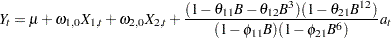The OUTEST= data set contains the values shown in Table 7.10.

 Obs _TYPE_ Y MU MA1_1 MA1_2 MA2_1 AR1_1 AR2_1 I1_1 I2_1 1 EST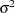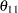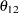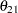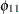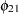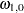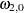2 STD . sesesesesesesese3 FACTOR . 0 1 1 2 1 2 1 1 4 LAG . 0 1 3 12 1 6 0 0 5 SHIFT . 0 0 0 0 0 0 0 0

Note that the symbols in the rows for _TYPE_=EST and _TYPE_=STD in Table 7.10 would be numeric values in a real data set.

Next, consider the following example:

```   proc arima data=input;
identify var=y cross=(x1 x2);
estimate p=1 q=1 input=(2 \$ (1)/(1,2)x1 1 \$ /(1)x2) outest=est;
run;

proc print data=est;
run;
```

The model specified by these statements is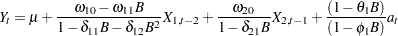The OUTEST= data set contains the values shown in Table 7.11.

 Obs _TYPE_ Y MU MA1_1 AR1_1 I1_1 I1_2 I1_3 I1_4 I2_1 I2_2 1 EST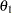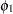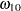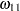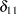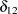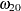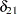2 STD . sesesesesesesesese3 FACTOR . 0 1 1 1 1 -1 -1 1 -1 4 LAG . 0 1 1 0 1 1 2 0 1 5 SHIFT . 0 0 0 2 2 2 2 1 1Previous Page | Next Page | Top of Page